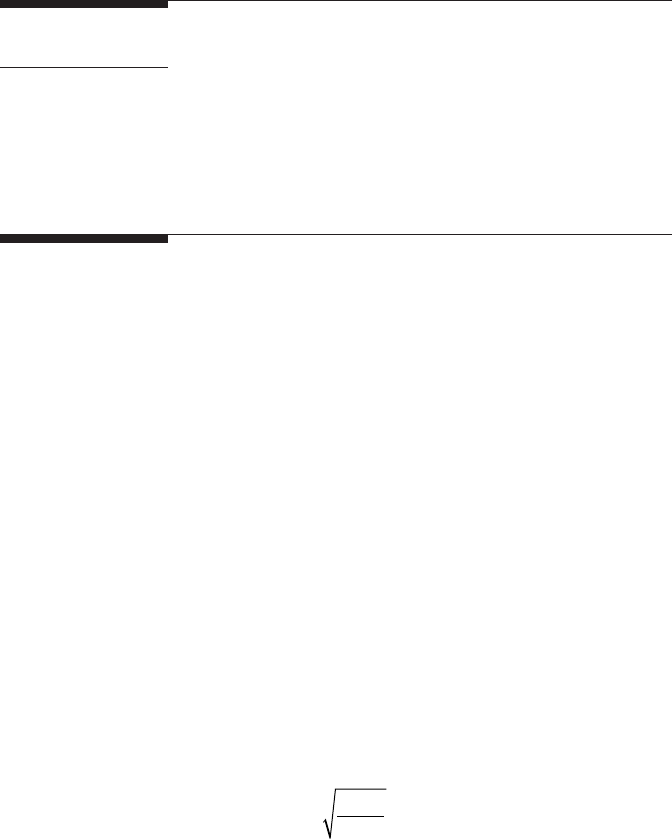53
7
Nanoindentation Techniques
Manjima Bhattacharya, Arjun Dey, and Anoop Kumar Mukhopadhyay
7.1 Introduction
7.1.1 Hardness Analysis
Based on the load versus depth-of-penetration data generated, the parameters
involved in a nanoindentation experiment (Figures7.1a and 7.1b) are given
by h
f
, the nal depth of penetration; h
max
, the maximum depth of penetration
of the indenter when the load P = P
max
; and h
c
, the contact depth, i.e., the dis-
placement where the indenter has maximum contact with the surface while
there can be two area concepts used in nanoindentation evaluation. If the area
is calculated from the contact depth, h
c
, it is generally denoted by the contact
area, A
s
. There is another area called A
p
, which is the projected contact area
of an ideal nanoindenter. Since the nanoindenters have very small tip radius,
typically about 40–200 nm, and the load may be ultralow, from a few microne-
wtons to a few millinewtons, it is expected that the indentation imprints also
will be very small. Therefore, to measure the contact area and the contact
height directly experimentally is rather difcult because of the elastic recov-
ery. Therefore, these are calculated based on the projected area, A
p
. Assuming
that a given nanoindenter (e.g., Vickers or Berkovich) has an ideal shape and
the surfaces that it indents are perfectly at, the contact depth (h
c
) is given by
=h
A
24.56
c
p
and when h
c
is known, the contact area A
c
is given by, e.g., A
c
= 26.44h
c
2
,
while the corresponding projected contact area A
p
is given by, e.g.,
A
p
(h
c
)=24.56h
c
2
. The quantity A
p
can be measured with sufcient accuracy
from the images ofthe nanoindent, provided that the tangent height is set
at typically about 90% below the surface height. Once A
p
is known, h
c
can
be calculated. When h
c
is calculated, the value of A
s
can be easily predicted,54 Nanoindentation of Brittle Solids
asmentionedpreviously. Because of the repeated contact events and friction
with the surface being indented, the tip of the nanoindenter gets worn out
and, as a result, it becomes rounded. Thereby, it physically shifts from its
ideal shape. Hence, the calibration of the indenter area function is done to
account for the deviation from the ideal shape.
Projected area:
()
=+++++Ah ah ah ah ah ah
pc 0c
2
1c 2c
1
2
3c
1
4
8c
1
128
(7.1)
Surface area:
()
=+++++Ah bh bh bh bh bh
sc 0c
2
1c 2c
1
2
3c
1
4
8c
1
128
(7.2)
The quantity a
0
in equation (7.1) is approximately 24.56, whereas the quantity
b
0
in equation (7.2) is approximately 26.44 . The calculations of the pro-
jected area functions are done through dedicated software packages (which
may vary from machine to machine) [3, 4] by analyzing the load–unload
curves generated from a series of nanoindents made over a range of prespec-
ied depths. Further, h
c
is related to h
f
by h
c
= h
f
(1 + e
c
), where e
c
physically is
about 10%–30% for most metals and reects the amount of elastic recovery
measured value of h
f
using standard software packages, h
c
is derived to digi-
tally reconstruct the fully loaded nanoindentation cavity surface from the
residual nanoindentation cavity surface (Figure7.2). To improve the accuracy
Residual Indent
Surface
Reconstructed
Indent Surface
FIGURE 7.2
Reconstructed nanoindent cavity surface and the residual nanoindent cavity surface.
Force
Pile-Ups
Displacement
(a) (b)
h
f
h
f
h
c
h
c
h
max
FIGURE 7.1
Denition of typical physical parameters in nanoindentation: (a) load (P) versus depth (h) plot;
(b) concept of contact depth (h
c
) and nal depth of penetration (h
f